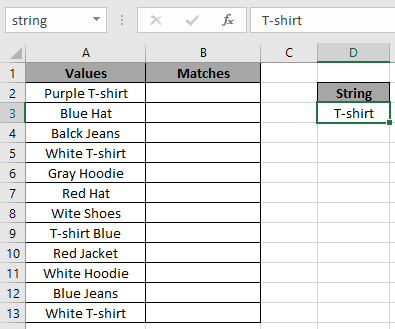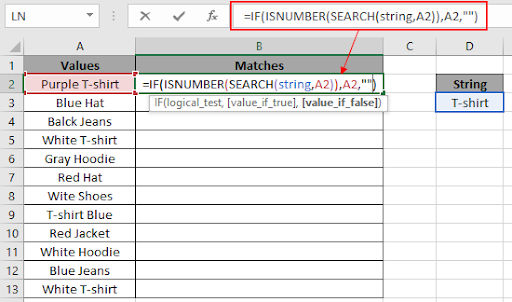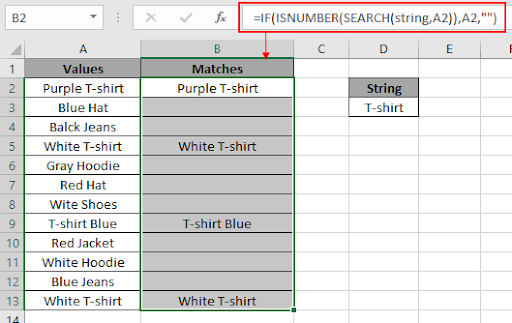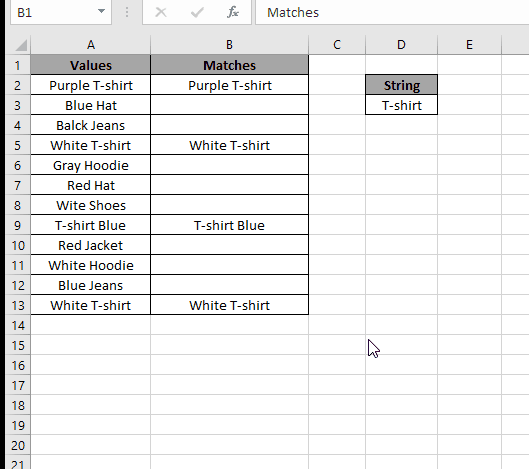# Partial matches in an array

In this article, we will learn about how to extract all partial matches in an array of values in Excel.

For instance, we have a large array of values and we need to extract all the values matching the string. We will use a combination of IF function, ISNUMBER function & SEARCH function. Let's get some understanding of these functions.

IF function is used for logic_test and returns value on the basis of the result of the test.

Syntax:

 = IF ( logical_test , [value_if_true] , [value_if_false] )

Excel’s ISNUMBER function is a boolean function that returns TRUE or FALSE if supplied value is a number or not respectively.

Syntax

 = ISNUMBER ( value )

SEARCH Function in Excel returns the position of the first character of the substring or search_text in a string or it could be fed into other function. SEARCH function is not case sensitive.

Syntax:

 = SEARCH ( find_text , within_text , [start_num] )

find_text : text to be found within_text.

within_text : find_text to be found in within_text.

[start_num] : character position to start from. Default is 1.

Now we will construct a formula using the above explained function. Use the formula:

FORMULA:

 = IF ( ISNUMBER ( SEARCH ( string , cell ) ) , cell , "" )

Explanation:

• SEARCH function finds the string in the cell value and returns the position of the string in the cell value if found or else it returns #VALUE! Error.
• ISNUMBER function checks if the SEARCH function returns number or not. ISNUMBER function returns TRUE if the returned value is True and returns FALSE for the #VALUE! Error.
• IF function returns cell value if ISNUMBER function returns TRUE and returns empty cell if the ISNUMBER function returns FALSE

Let’s understand this function using it in an example.

Here we have a list of values and a string value ( T-shirt ).We need to find out the list of matches in Matches column. Use the below formula to extract all partial matches for the string ( T-shirt ).

Use the formula:

 = IF ( ISNUMBER ( SEARCH ( string , A2 ) ), A2 , "" )

Explanation:

• SEARCH function finds the string in the cell value and returns the 8 as the  position of the string ( T-shirt ) in the cell value ( Purple T-shirt ).
• ISNUMBER function checks the value 8 is a number or not. ISNUMBER function returns TRUE.
• IF function returns value_if_True which is the cell value ( Purple T-shirt ).Here cell value is given as cell reference. Copy the formula to the rest of the cells using the Ctrl + D shortcut key or drag down option in excel.As you can see we got all the values using the formula. Now we need a list out of the Matches. So for that we will filter results. Follow the below steps to filter results.

1. Use the Ctrl + Shift + L option to apply filter
2. Right click the arrow key on the Matches field.
3. Untick the (blanks) option and click OK.As you can see from the above gif to extract the list out of the matches values.
Hope you understood how to use partial matches in an array in Excel. Explore more articles on Excel text function here. Please feel free to state your query or feedback for the above article.
Related Articles

How to Remove Text in Excel Starting From a Position

Validation of text entries

Create drop down list in excel with colour

Remove leading and trailing spaces from text in Excel

How to use the LARGE function in Excel

How to use the SMALL function in Excel

How to use Wildcards in Excel
Popular Articles

50 Excel Shortcut to Increase Your Productivity

Edit a dropdown list

Absolute reference in Excel

If with conditional formatting

If with wildcards

Vlookup by date

Convert Inches To Feet and Inches in Excel 2016

Join first and last name in excel

Count cells which match either A or B

Terms and Conditions of use

The applications/code on this site are distributed as is and without warranties or liability. In no event shall the owner of the copyrights, or the authors of the applications/code be liable for any loss of profit, any problems or any damage resulting from the use or evaluation of the applications/code.